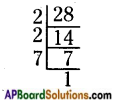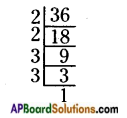# AP Board 6th Class Maths Solutions Chapter 3 HCF and LCM Ex 3.4

AP State Syllabus AP Board 6th Class Maths Solutions Chapter 3 HCF and LCM Ex 3.4 Textbook Questions and Answers.

## AP State Syllabus 6th Class Maths Solutions 3rd Lesson HCF and LCM Ex 3.4

Question 1.
Prepare a factor tree for 90.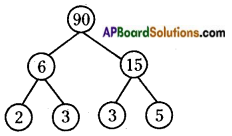Prime factorization of 90 is 2 × 3 × 3 × 5 = 90Question 2.
Factorise 84 by division method.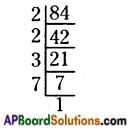Prime factorization of 84 is 2 × 2 × 3 × 7 = 84

Question 3.
Write the greatest 4 digit number and express it in the form of its prime factors.
The greatest 4 – digit number is 9999.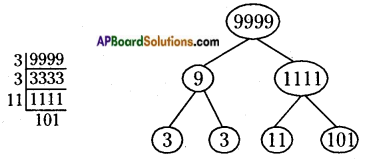Prime factorization of 9999 is 3 × 3 × 11 × 101 = 9999

Question 4.
Write the prime factorization of 96 by factor tree method.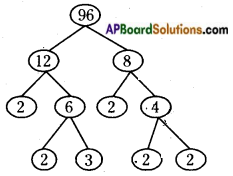Prime factorization of 96 is 2 × 2 × 3 × 2 × 2 × 2 = 96Question 5.
I am the smallest number, having four different prime factors. Can you find me?